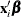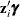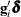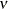The COUNTREG Procedure

OUTPUT OUT= Data Set

The OUTPUT statement creates a new SAS data set that contains all the variables in the input data set and, optionally, the estimates of, the expected value of the response variable, and the probability that the response variable will take the current value or other values that you specify. In a zero-inflated model, you can also request that the output data set contain the estimates of, and the probability that the response is zero as a result of the zero-generating process. In a Conway-Maxwell-Poisson model, you can also request that the output data set contains estimates of,,,, mode, variance and dispersion.

Except for the probability of the current value, these statistics can be computed for all observations in which the regressors are not missing, even if the response is missing. By adding observations that have missing response values to the input data set, you can compute these statistics for new observations or for settings of the regressors that are not present in the data without affecting the model fit.